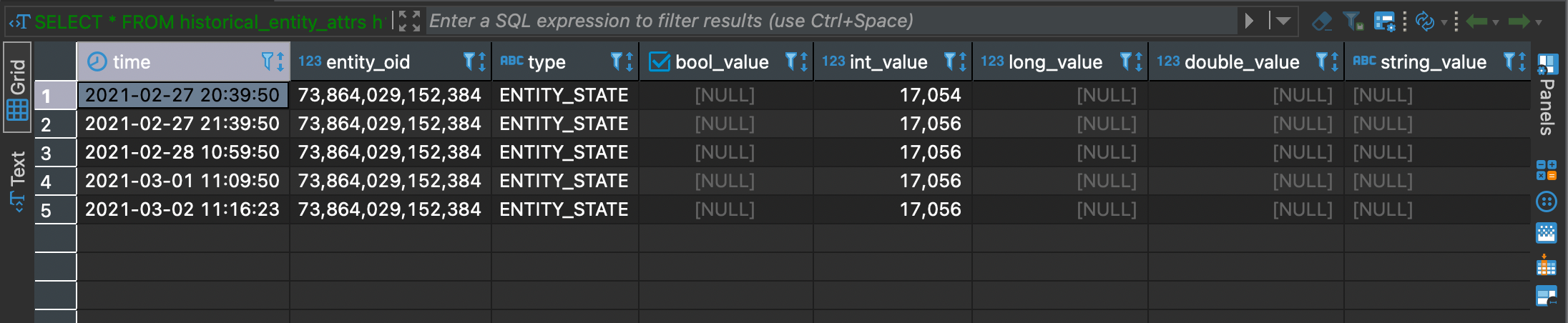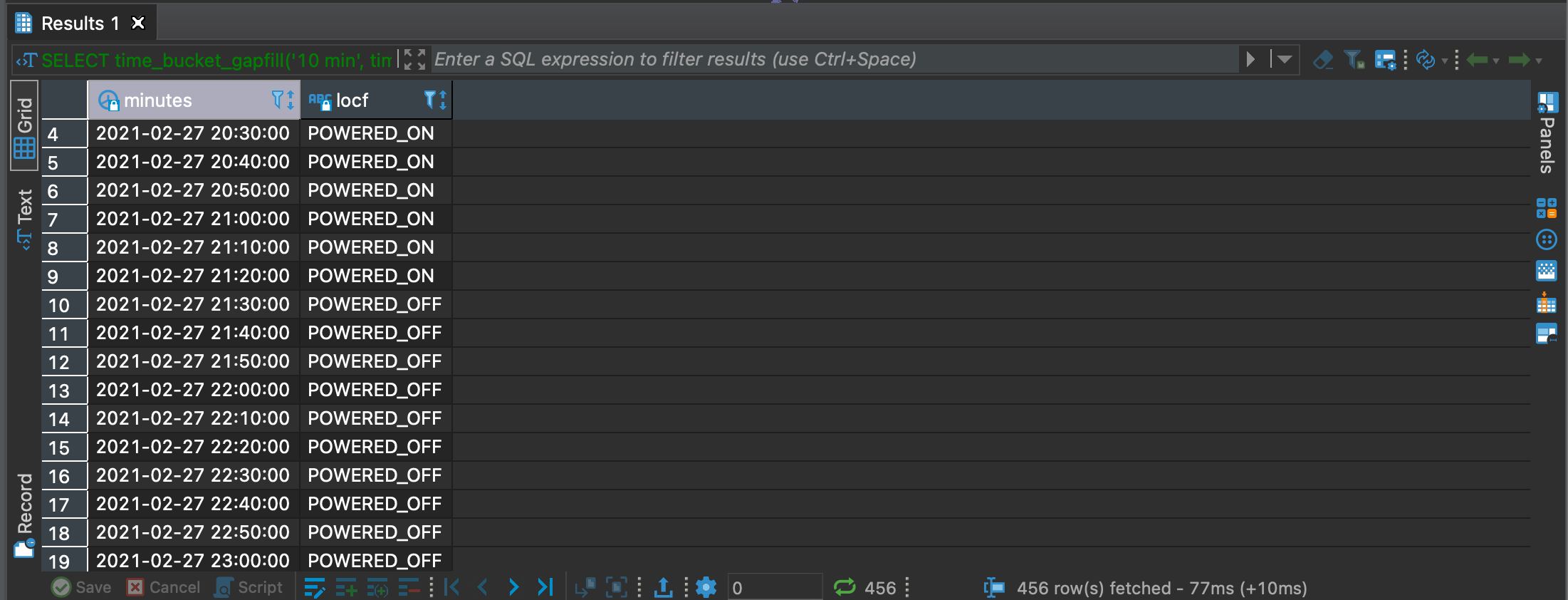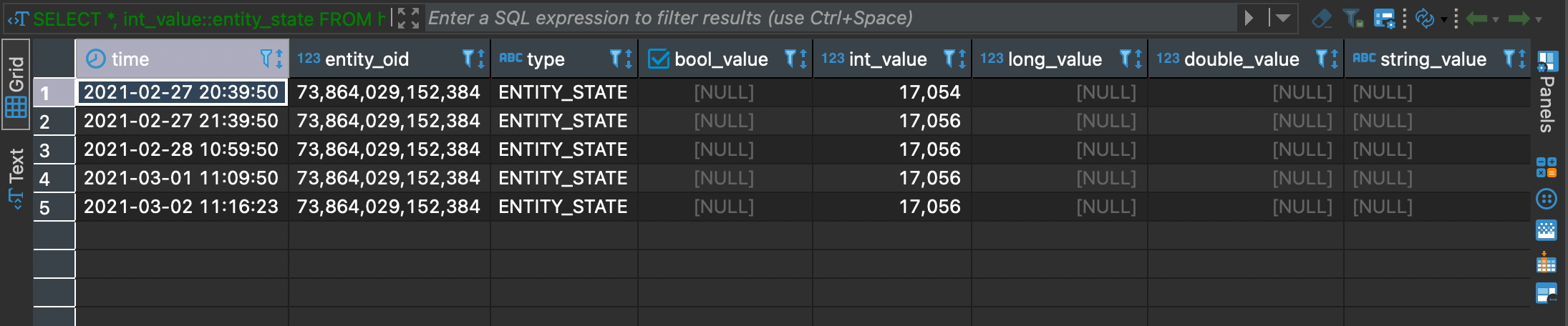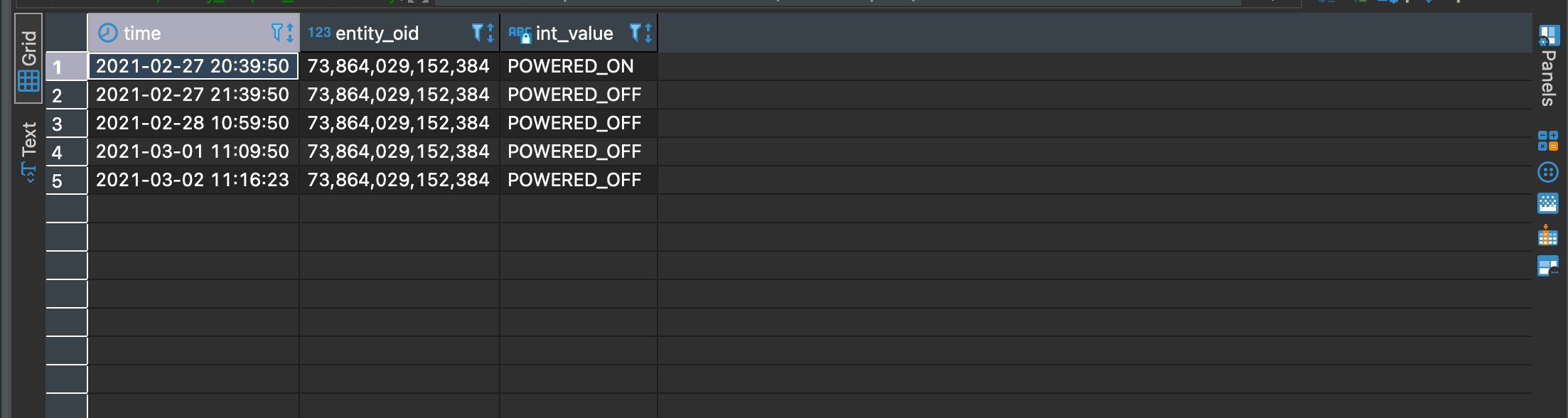# historical_entity_attrs Table

This table tracks the historical attributes associated with an entity. Each row represents the value of a particular attribute of an entity at a particular point in time. New rows are written when the value of a property changes between topologies, and at regular intervals (e.g. daily) to keep a recent value available within a reasonable time range.

historical_entity_attrs

## historical_entity_attrs table

Column Name

Type

Nullable

Primary

Description

time

timestamp with time zone

false

false

The time this attribute was recorded.

entity_oid

bigint

false

false

The oid of the entity the attribute pertains to.

type

attr_type

false

false

The attribute type.

bool_value

boolean

true

false

If the attribute is boolean, this is the boolean value.

int_value

int

true

false

If the attribute is an integer, this is the integer value. If the attribute is an enum, this is the oid of the enum in the pg_enum table (to guard against name or order changes). The int_value should be castable to the enum via a custom cast (e.g. int _value::entity_state = 'POWERED_ON').

long_value

bigint

true

false

If the attribute is a long, this is the long value.

double_value

double precision

true

false

If the attribute is a double, this is the double value.

string_value

text

true

false

If the attribute is a string, this is the string value.

int_arr_value

int[]

true

false

If the attribute is a list of ints, this is the value. If the attribute is a list of enums, these are the OIDs of the enums in the pg_enum table (to guard against name or order changes).

long_arr_value

bigint[]

true

false

If the attribute is a list of longs, this is the value.

string_arr_value

text[]

true

false

If the attribute is a list of strings, this is the value.

double_arr_value

float8[]

true

false

If the attribute is a list of doubles, this is the value.

json_value

jsonb

true

false

If the attribute is a JSON object, this is the value.

## Sample Use Cases

### Get All Powered On Entities:

This query returns all the entities in the historical_entity_attrs that do not have a powered_off state given a certain time range.

``````select *
from historical_entity_attrs h1
where h1.type = 'ENTITY_STATE' and h1.int_value::entity_state != 'POWERED_OFF' AND h1.time between \$__timeFrom() and \$__timeTo()
``````

### Gap-filling data:

Data in this table are not written at every broadcast. Because of this, if we ask for those data in a time range, we might only get few data points. Consider this query in which we get the values for one entity in a time range:

``````SELECT * FROM historical_entity_attrs h1
where h1.type = 'ENTITY_STATE'
and entity_oid ='73864029152384'
and time between (timestamptz '2021-03-01T01:00:00Z' - interval '24 hour') and '2021-03-03T04:59:59Z'
order by time;
``````In order to solve this problem we use a timescaledb function that fills the time ranges with data, as if those data were written at a constant interval. This means that if we want data every interval `t` but we only have data at `t1` and `t4`, with this function we can generate data at `t2` and `t3` with the same value of `t1`. The function that we use is called `time_bucket_gapfill` and here’s a sample query. Notice how in the result we have data points at a constant interval of 10 minutes:

``````SELECT
time_bucket_gapfill('10 min', time) AS minutes,
locf(max(h1.int_value::entity_state))
FROM historical_entity_attrs h1
where h1.type = 'ENTITY_STATE'
--     AND h1.int_value::entity_state = 'POWERED_ON'
and entity_oid ='73864029152384'
and time between (timestamptz '2021-03-01T01:00:00Z' - interval '24 hour') and '2021-03-03T04:59:59Z'
GROUP BY minutes
``````### Casting Entity States to Integers:

Entity States are internally represented as integers. This means that a property such as `POWERED_OFF` is stored as a number. In order to work with this the queries need to cast the integer to the corresponding entity state. This is done with the following cast, supported by Postgres: `int_value::entity_state `. So for example, take a look at the two queries below, the first one without the cast, and the second query with it:

``````SELECT time, entity_oid, entity_state FROM historical_entity_attrs h1
where h1.type = 'ENTITY_STATE'
and entity_oid ='73864029152384'
and time between (timestamptz '2021-03-01T01:00:00Z' - interval '24 hour') and '2021-03-03T04:59:59Z'
order by time;
````````````SELECT time, entity_oid, int_value::entity_state FROM historical_entity_attrs h1
where h1.type = 'ENTITY_STATE'
and entity_oid ='73864029152384'
and time between (timestamptz '2021-03-01T01:00:00Z' - interval '24 hour') and '2021-03-03T04:59:59Z'
order by time;
``````